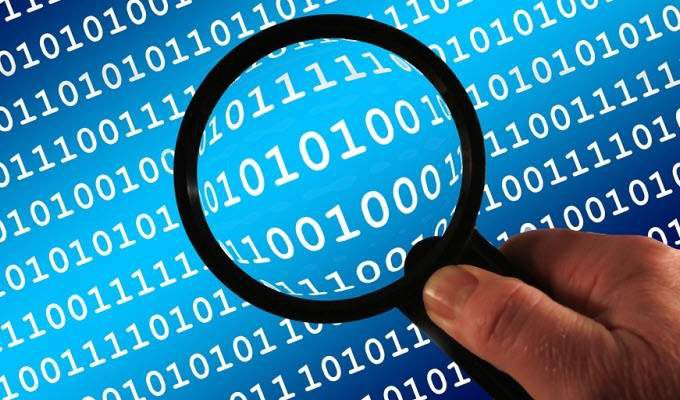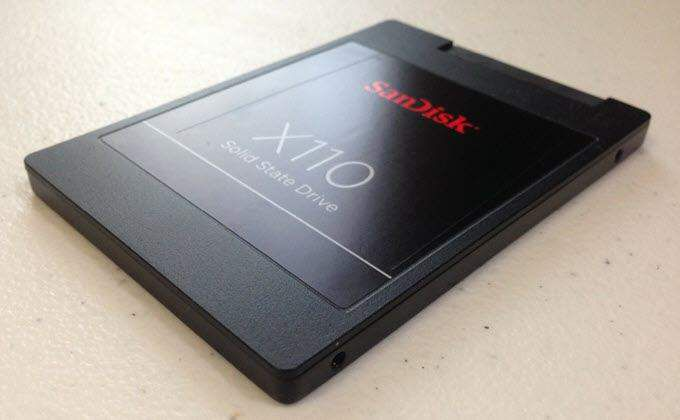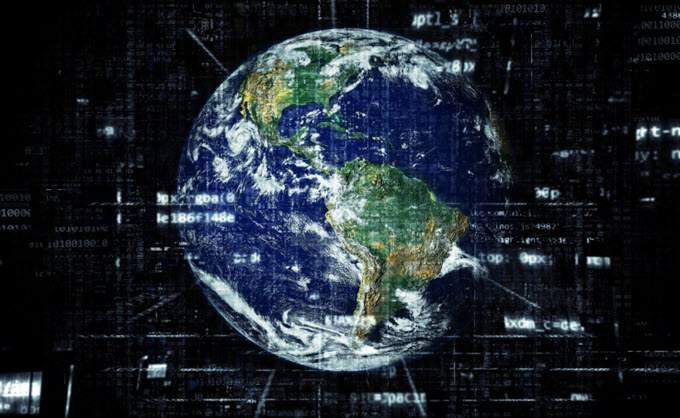# Computer help - file server

[ Blog ] - [ File Server ] - [ Удаленная компьютерная помощь ]

If you’re new to computers (or even if you’re not), the names that get applied to different memory sizes can seem strange.

Whether you’re talking about an 8-megabyte memory card, a 500-gigabyte hard drive, or a 1 terabyte SSD drive, the terms always seem abstract and random.

How exactly do you gauge just how much space a gigabyte, a terabyte, or even a petabyte describes? What Is a Byte?

To understand how the larger blocks of memory work, it’s important to build an appreciation for the smaller blocks of space that those larger ones are made from.

In simple terms, a single byte is typically eight binary digits. A binary digit is a 1 or a 0, which in very old computers literally represented a switch that was on or off.

There are some computer systems that have bytes of other lengths, but most modern computers today are based on an eight-bit byte binary system.Those eight bits (a byte) usually represent a character like a letter or number. Bytes can also represent symbols that represent one piece of a larger object like an image.

Since a “byte” is the smallest unit of data, then other names are needed for larger units of data made up of even more bits. The important thing to keep in mind is that all the larger units are made up of a fixed number of bytes, and each byte typically contains eight bits.

As you start stacking up more bytes, you can determine the name of the unit based on the number of bytes. A Kilobyte is 1,024 Bytes

You would think that since the prefix “kilo” typically means 1,000, that kilobyte would have 1,000 bytes.

The reality is that since computers store data using the binary system, and the binary system is based on powers of 2, the actual number of bytes is 1,024.

You can see this when you look at how the power of 2’s works. 2^0 = 1 2^1 = 2 2^2 = 4 2^3 = 8 2^4 = 16 2^5 = 32 2^6 = 64 2^7 = 128 2^8 = 256 2^9 = 512 2^10 = 1024

The first binary value that represents 1,000 bytes is 1,024. Therefore, a kilobyte contains 1,024 bytes.

You can estimate the size that information would require based on the number of characters in that data. Take a 200-page book as an example. Typically, each page in a book has about 300 word per page. That means the entire book is about 60,000 words.An average word is about 6 characters. That means a 60,000-word book has about 360,000 characters.

To store this book electronically would require 360,000 bytes.

You can represent this in kilobytes (KB) by dividing 360,000 bytes by 1024. This means a 60,000-word book would require about 351.56 kilobytes of digital storage. What is a Gigabyte?

In the metric system, the prefix “Giga” means a unit of measure of 10 to the power of 9, or 1,000,000,000. But remember, to represent this in the computer binary system, it needs to take the binary factor of 2’s into account.

So, working up to Gigabyte using power of 2’s, we`ll need to go all the way to 2^30 to get the first number over 1 billion, which is 1,073,741,824 bytes.

So far you know that a kilobyte is 1,024 bytes. What about everything between 1,024 and1,073,741,824 ? Kilobyte (KB): A thousand bytes, or a kilobyte, is 1,024 bytes. Megabyte (MB): A million bytes, or a megabyte, is represented as 1,024 kilobytes. Gigabyte (GB): A billion bytes, or a gigabyte, is represented as 1,024 megabytes.

To put the size of a gigabyte into perspective, consider that a single gigabyte can store about 230 music tracks, or almost 600 five-megapixel photographs. You could even store a standard 1.5-hour movie on 1 gigabyte. What Is a Terabyte?What is the next power of 10 number greater than a billion? That would be a trillion.

The prefix for a trillion is “tera”. A terabyte is 10 to the power of 12 bytes, represented in binary.

That means 1 terabyte (TB) is 1024 gigabytes. Most modern hard drives store half of this amount of data. A terabyte, a trillion bytes, is a lot of information.

In recent years, manufacturers have started releasing new computers with a one or two terabyte drives. It would be very difficult for any user to fill up such a hard drive, unless they’re producing many hours of high-definition video every day.

Consider that a standard floppy drive in the 1990’s could hold only thousands of bytes. A CD-ROM could store 700 megabytes, and a DVD-ROM could store 4.7 GB. But the hard drives of today can store trillions of bytes. A 1 terabyte drive could store 217 DVD-ROM’s worth of data. We’ve come a long way. What Is a Petabyte?The next storage unit to consider is what’s known as a petabyte.

The prefix “peta” is the measurement unit for one quadrillion, or 10 to the power of 15.

Since this is 1,000 units of one trillion (tera), then one petabyte is equivalent to 1,024 terabytes. That’s one quadrillion bytes.

You would think this volume of information could never be used. However, there are petabytes of information flowing through computer systems and networks today, however hard that may be to believe.

But consider the following modern applications of petabyte sized technology: Google processes over 24 petabytes of information every day. Mobile phone networks transmit over 20 petabytes to and from users every day. The Blue Waters supercomputer has over 500 petabytes of tape storage. The United States Library of Congress contains over 7 petabytes of digital data in its archives. World of Warcraft servers require over 1.5 petabytes of storage to run its online game.

The scale of a petabyte is hard to wrap your head around, but once you consider the scenarios above, it becomes quite clear just how much data is involved.

A single petabyte could store over 10,000 hours of television programming. If you filled an entire four-drawer filing cabinet with documents filled with text, you could fit 20 million of those file cabinets into a petabyte.

In fact, you could store every single written manuscript created by humanity since the beginning of recorded history in 50 petabytes.

That’s a lot of data. Understanding Memory Terminology

It’s important to understand the units of memory because it’s used everywhere where there’s technology these days. Any time you buy a computer, a mobile phone, or a tablet, the specifications are all written in terms of memory storage, and how much data the technology can transmit.

If you understand all these terms, then you’ll know just how much better one computer is than other. You’ll appreciate how much better a 4G mobile network is than a 3G one. You’ll appreciate how much more you’ll be able to store on a 1 terabyte memory card rather than a 500 megabyte one.

As technology continues to advance, it’s possible there will be new units of memory to learn about. But for now, these terms are all you’ll need to know.

And if you`ve gotten this far, you should jump over to an article we`ve written about understanding network transfer speeds, which consists of megabits per second, gigabits per second, etc. This will help you understand when your ISP tells you that your download speed is 15 MBps. Enjoy!

SETUP UA COMPUTER BLOG